# RD Sharma Solutions for Class 6 Maths Chapter 6: Fractions Exercise 6.8

Exercise 6.8 of Chapter 6 contains problems which are based on addition and subtraction of ‘like fractions’. Students can use the RD Sharma Solutions Class 6 prepared by faculty at BYJU’S in order to improve their speed in solving problems accurately. The solutions are solved based on the RD Sharma textbook of current CBSE syllabus. The students can self analyse the concepts which require more time to understand and work on them. To get a brief knowledge about the important concepts which are covered under this exercise, the students can download PDF of RD Sharma Solutions for Class 6 Maths Chapter 6 Fractions Exercise 6.8 here.

## RD Sharma Solutions for Class 6 Maths Chapter 6: Fractions Exercise 6.8 Download PDF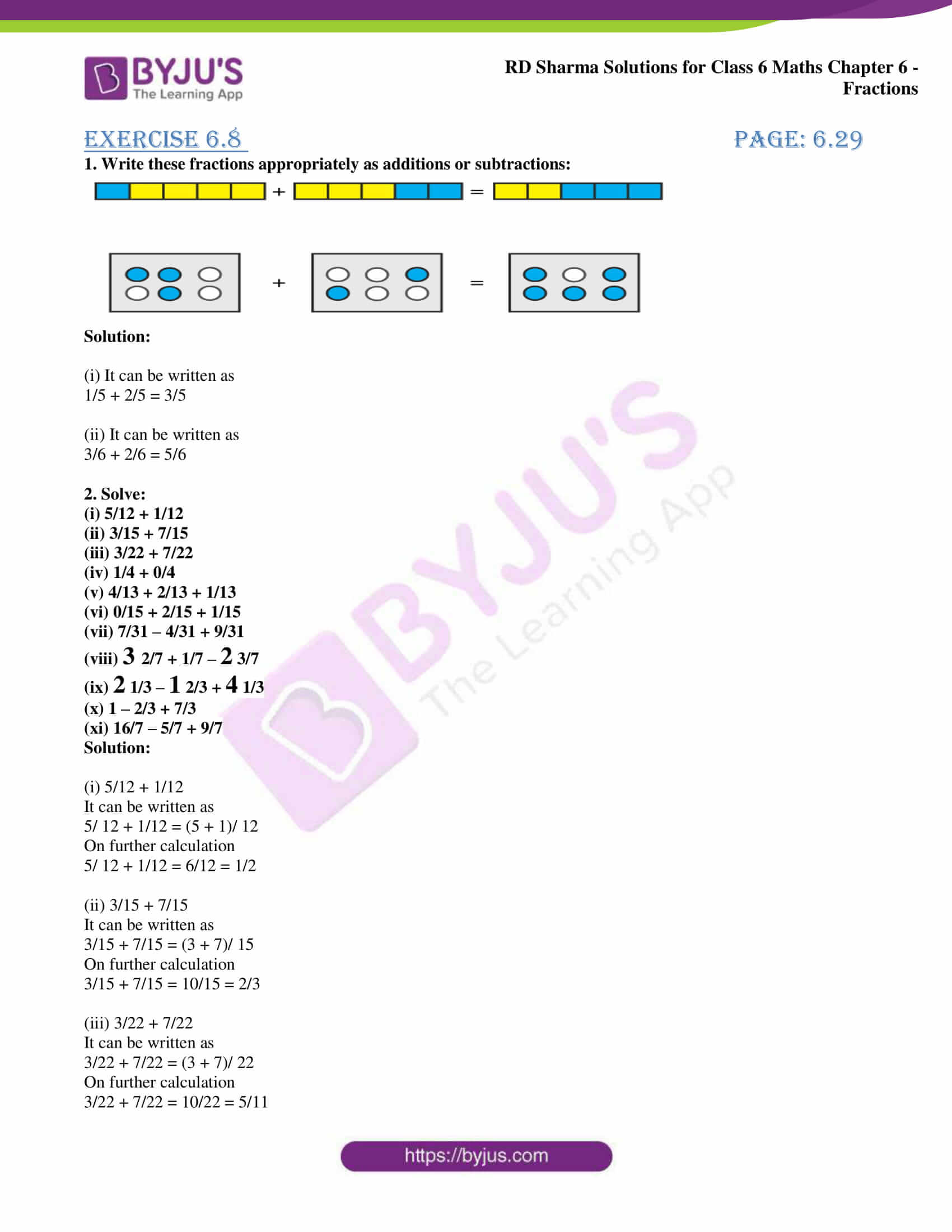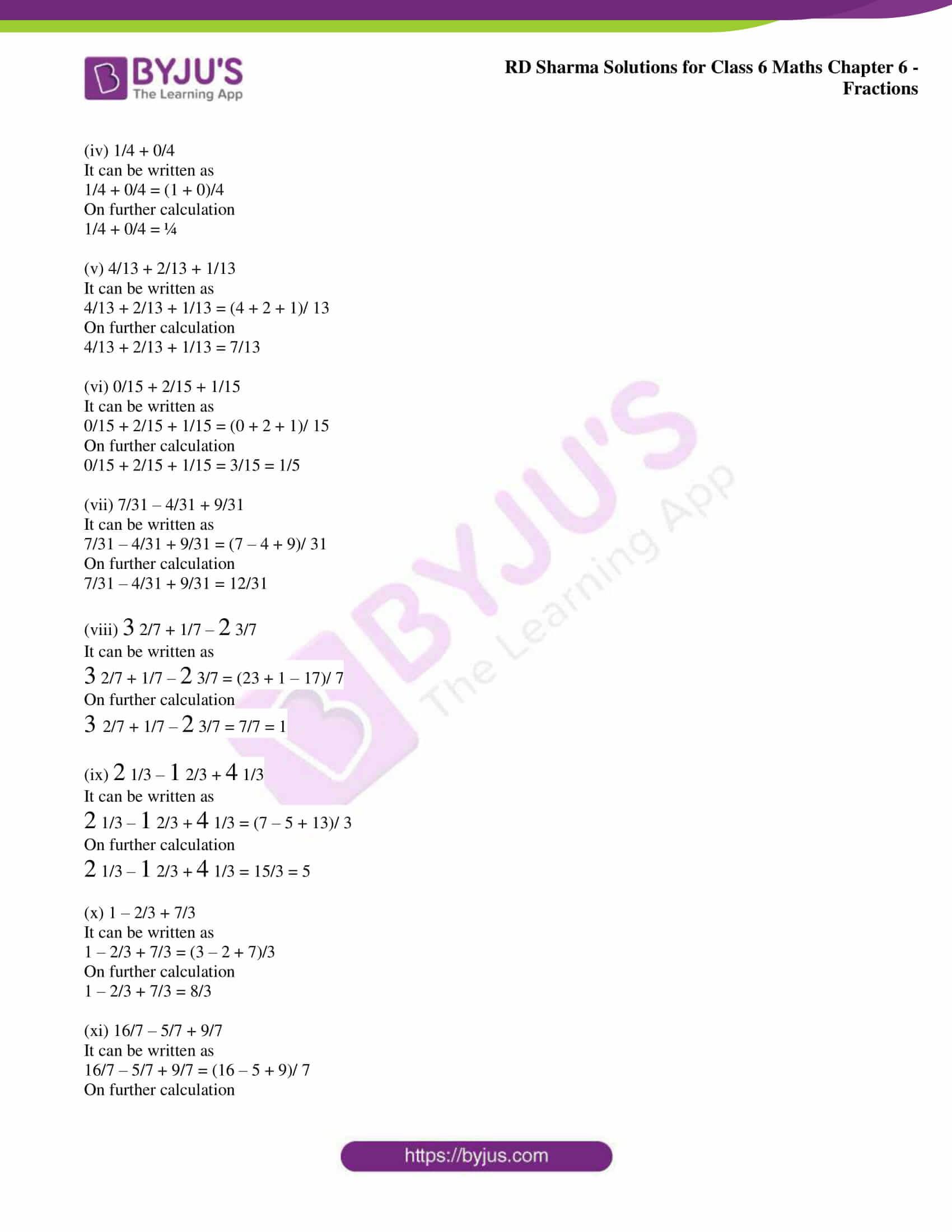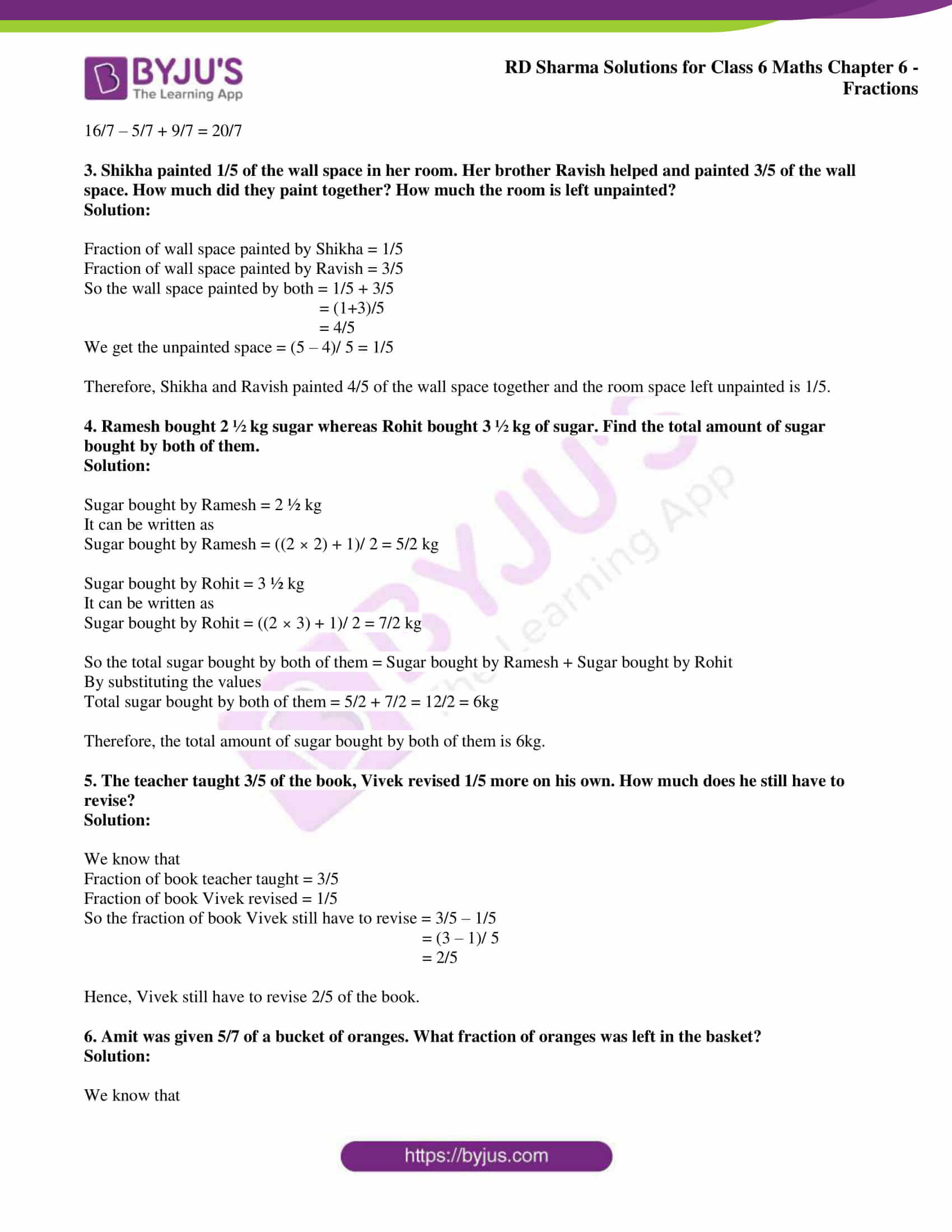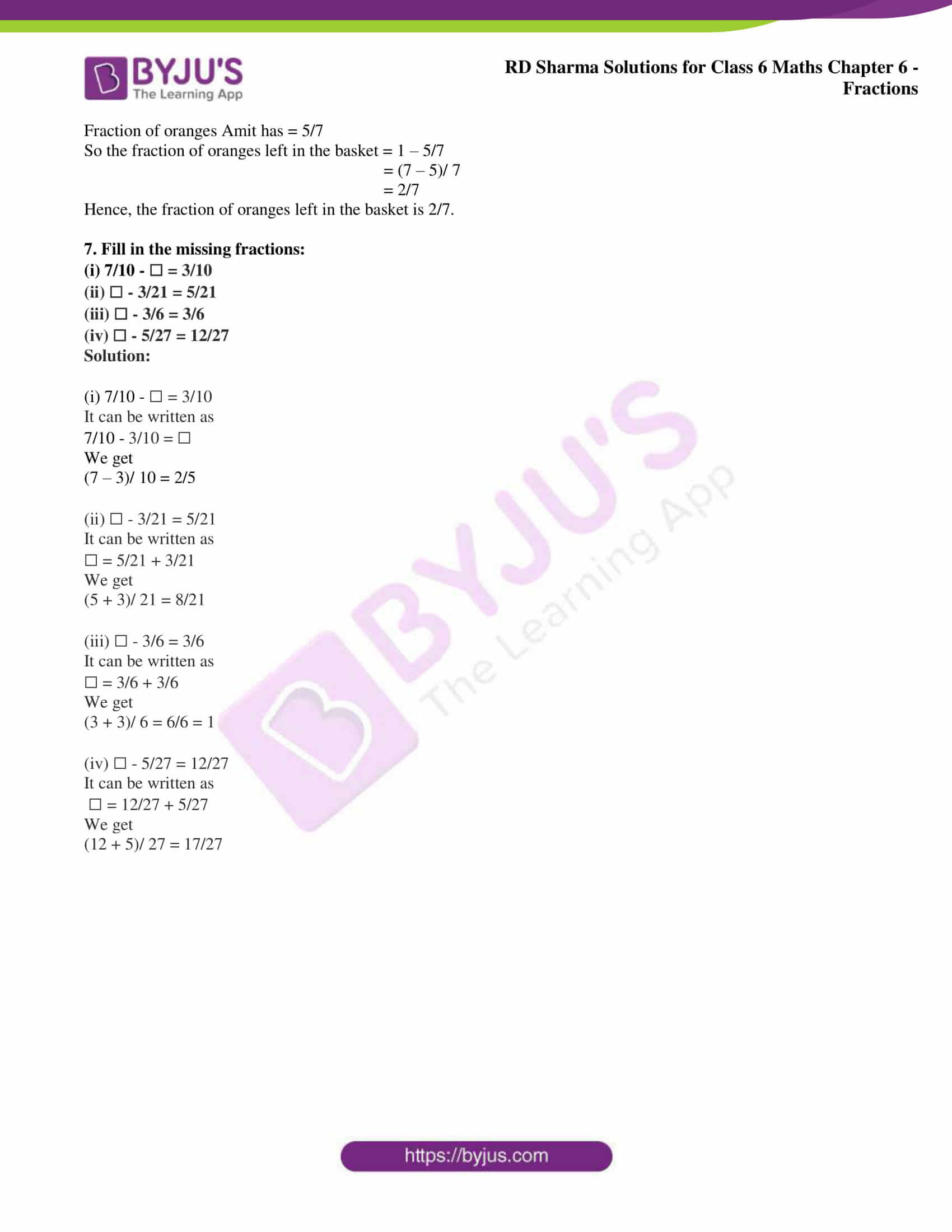### Access answers to Maths RD Sharma Solutions for Class 6 Chapter 6: Fractions Exercise 6.8

1. Write these fractions appropriately as additions or subtractions: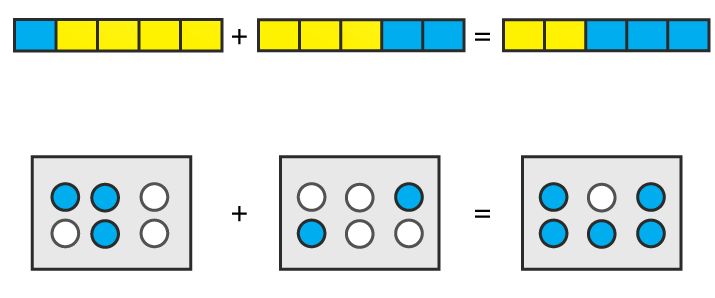Solution:

(i) It can be written as

1/5 + 2/5 = 3/5

(ii) It can be written as

3/6 + 2/6 = 5/6

2. Solve:

(i) 5/12 + 1/12

(ii) 3/15 + 7/15

(iii) 3/22 + 7/22

(iv) 1/4 + 0/4

(v) 4/13 + 2/13 + 1/13

(vi) 0/15 + 2/15 + 1/15

(vii) 7/31 – 4/31 + 9/31

(viii) 3 2/7 + 1/7 – 2 3/7

(ix) 2 1/3 – 1 2/3 + 4 1/3

(x) 1 – 2/3 + 7/3

(xi) 16/7 – 5/7 + 9/7

Solution:

(i) 5/12 + 1/12

It can be written as

5/ 12 + 1/12 = (5 + 1)/ 12

On further calculation

5/ 12 + 1/12 = 6/12 = 1/2

(ii) 3/15 + 7/15

It can be written as

3/15 + 7/15 = (3 + 7)/ 15

On further calculation

3/15 + 7/15 = 10/15 = 2/3

(iii) 3/22 + 7/22

It can be written as

3/22 + 7/22 = (3 + 7)/ 22

On further calculation

3/22 + 7/22 = 10/22 = 5/11

(iv) 1/4 + 0/4

It can be written as

1/4 + 0/4 = (1 + 0)/4

On further calculation

1/4 + 0/4 = ¼

(v) 4/13 + 2/13 + 1/13

It can be written as

4/13 + 2/13 + 1/13 = (4 + 2 + 1)/ 13

On further calculation

4/13 + 2/13 + 1/13 = 7/13

(vi) 0/15 + 2/15 + 1/15

It can be written as

0/15 + 2/15 + 1/15 = (0 + 2 + 1)/ 15

On further calculation

0/15 + 2/15 + 1/15 = 3/15 = 1/5

(vii) 7/31 – 4/31 + 9/31

It can be written as

7/31 – 4/31 + 9/31 = (7 – 4 + 9)/ 31

On further calculation

7/31 – 4/31 + 9/31 = 12/31

(viii) 3 2/7 + 1/7 – 2 3/7

It can be written as

3 2/7 + 1/7 – 2 3/7 = (23 + 1 – 17)/ 7

On further calculation

3 2/7 + 1/7 – 2 3/7 = 7/7 = 1

(ix) 2 1/3 – 1 2/3 + 4 1/3

It can be written as

2 1/3 – 1 2/3 + 4 1/3 = (7 – 5 + 13)/ 3

On further calculation

2 1/3 – 1 2/3 + 4 1/3 = 15/3 = 5

(x) 1 – 2/3 + 7/3

It can be written as

1 – 2/3 + 7/3 = (3 – 2 + 7)/3

On further calculation

1 – 2/3 + 7/3 = 8/3

(xi) 16/7 – 5/7 + 9/7

It can be written as

16/7 – 5/7 + 9/7 = (16 – 5 + 9)/ 7

On further calculation

16/7 – 5/7 + 9/7 = 20/7

3. Shikha painted 1/5 of the wall space in her room. Her brother Ravish helped and painted 3/5 of the wall space. How much did they paint together? How much the room is left unpainted?

Solution:

Fraction of wall space painted by Shikha = 1/5

Fraction of wall space painted by Ravish = 3/5

So the wall space painted by both = 1/5 + 3/5

= (1+3)/5

= 4/5

We get the unpainted space = (5 – 4)/ 5 = 1/5

Therefore, Shikha and Ravish painted 4/5 of the wall space together and the room space left unpainted is 1/5.

4. Ramesh bought 2 ½ kg sugar whereas Rohit bought 3 ½ kg of sugar. Find the total amount of sugar bought by both of them.

Solution:

Sugar bought by Ramesh = 2 ½ kg

It can be written as

Sugar bought by Ramesh = ((2 × 2) + 1)/ 2 = 5/2 kg

Sugar bought by Rohit = 3 ½ kg

It can be written as

Sugar bought by Rohit = ((2 × 3) + 1)/ 2 = 7/2 kg

So the total sugar bought by both of them = Sugar bought by Ramesh + Sugar bought by Rohit

By substituting the values

Total sugar bought by both of them = 5/2 + 7/2 = 12/2 = 6kg

Therefore, the total amount of sugar bought by both of them is 6kg.

5. The teacher taught 3/5 of the book, Vivek revised 1/5 more on his own. How much does he still have to revise?

Solution:

We know that

Fraction of book teacher taught = 3/5

Fraction of book Vivek revised = 1/5

So the fraction of book Vivek still have to revise = 3/5 – 1/5

= (3 – 1)/ 5

= 2/5

Hence, Vivek still have to revise 2/5 of the book.

6. Amit was given 5/7 of a bucket of oranges. What fraction of oranges was left in the basket?

Solution:

We know that

Fraction of oranges Amit has = 5/7

So the fraction of oranges left in the basket = 1 – 5/7

= (7 – 5)/ 7

= 2/7

Hence, the fraction of oranges left in the basket is 2/7.

7. Fill in the missing fractions:

(i) 7/10 – ☐ = 3/10

(ii) ☐ – 3/21 = 5/21

(iii) ☐ – 3/6 = 3/6

(iv) ☐ – 5/27 = 12/27

Solution:

(i) 7/10 – ☐ = 3/10

It can be written as

7/10 – 3/10 = ☐

We get

(7 – 3)/ 10 = 2/5

(ii) ☐ – 3/21 = 5/21

It can be written as

☐ = 5/21 + 3/21

We get

(5 + 3)/ 21 = 8/21

(iii) ☐ – 3/6 = 3/6

It can be written as

☐ = 3/6 + 3/6

We get

(3 + 3)/ 6 = 6/6 = 1

(iv) ☐ – 5/27 = 12/27

It can be written as

☐ = 12/27 + 5/27

We get

(12 + 5)/ 27 = 17/27